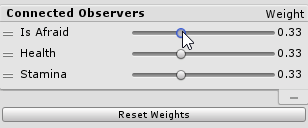# Applying Weights to Conditions

Every connection between an Observer and a Decision contains a Condition. By default, each Condition equally contributes to their corresponding Decision(s). To modify the weight of each Condition we can click on the Decision and move the sliders in the inspector.

These sliders act like the sliders on the Humble Bundle contributions page. When you increase one of the sliders, the other sliders will decrease proportionally. The total of all weights is always ~1. Each Condition takes a percentage of the max score that can be generated for the Decision.

In the table below, we state that our first Condition Weight 1 can only generate at most a subscore of 0.3. Condition Weight 2 can only generate at most a subscore of 0.7. Our Total Subscore can be at most ~1 and is multiplied by our Decision’s Priority.

Our potential score follows the formula:

`Potential Total Score with Weights = SUM(all_condition_subscores) * Priority`

Condition Weight 1 Subscore 1 Condition Weight 2 Subscore 2 Total Subscore Priority Potential Total Score with Weights
0.3 0 0.7 0.7 0.7 3 2.1 = ((0 + 0.7) * 3)

NOTE: We try to accurately display information, but due to limitations of floating point precision, we treat that 0.33 + 0.33 + 0.33 as 1.

In the event we would like to restore our default weights, a Reset Weights button is provided in the inspector. This equally distributes the Conditions’ weights.